0收藏

# 1 类继承

Python 是面向对象的编程语言，因此支持面向对象的三大特性之一：继承。

``````Python 3.10.4 (v3.10.4:9d38120e33, Mar 23 2022, 17:29:05) [Clang 13.0.0 (clang-1300.0.29.30)] on darwin
>>> dir(object)
['__class__', '__delattr__', '__dir__', '__doc__', '__eq__', '__format__', '__ge__', '__getattribute__', '__gt__', '__hash__', '__init__', '__init_subclass__', '__le__', '__lt__', '__ne__', '__new__', '__reduce__', '__reduce_ex__', '__repr__', '__setattr__', '__sizeof__', '__str__', '__subclasshook__']
>>>
``````

Python 中继承类的语法如下：

``````class SubClass(SuperClass):
``````

## 1.1 继承的简单例子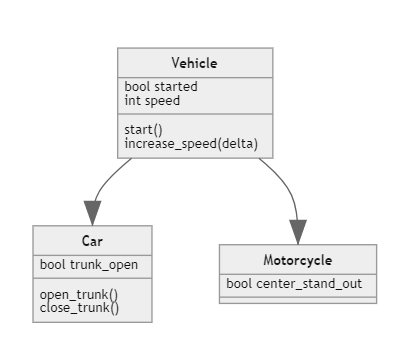Python 代码实现如上继承关系，如下：

``````class Vehicle:

def __init__(self, started =  False, speed = 0):
self.started = started
self.speed = speed

def start(self):
self.started = True
print("启动，皮皮虾我们走")

def stop(self):
self.speed = 0

def increaseSpeed(self, delta):
if self.started:
self.speed += delta
print("Vroooooom!")
else:
print("还没启动呢！")

class Car(Vehicle):
trunk_open = False
def open_trunk(self):
self.trunk_open = True
def close_trunk(self):
self.trunk_open = False
``````

``````class Motorcycle(Vehicle):
def __init__(self, center_start_out = False):
self.center_start_out = center_start_out
super().__init__()
def start(self):
print("Sorry, out of fuel!")
``````

``````class Vehicle:

def __init__(self, started =  False, speed = 0):
self.started = started
self.speed = speed

def start(self):
self.started = True
print("启动，皮皮虾我们走")

def stop(self):
self.speed = 0

def increaseSpeed(self, delta):
if self.started:
self.speed += delta
print("Vroooooom!")
else:
print("还没启动呢！")

class Car(Vehicle):
trunk_open = False
def open_trunk(self):
self.trunk_open = True
def close_trunk(self):
self.trunk_open = False

class Motorcycle(Vehicle):
def __init__(self, center_start_out = False):
self.center_start_out = center_start_out
super().__init__()

V = Vehicle()
C = Car()
M = Motorcycle()

print(type(V))
print(type(C))
print(type(M))

print("摩托车是交通工具的实例吗？",isinstance(M, Vehicle))
``````

`super()` 函数返回一个代表父类的临时对象。这用于访问父类的方法和属性。在 `super()` 函数的帮助下，我们还可以访问被覆盖的方法。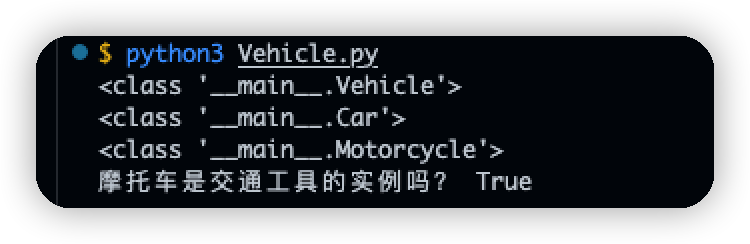# 2 继承的类型

## 2.1 单继承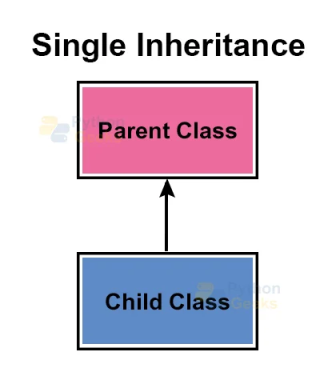``````class Animal:
print("我是动物")

class Cat(Animal):
print("我是猫")
``````

## 2.2 多继承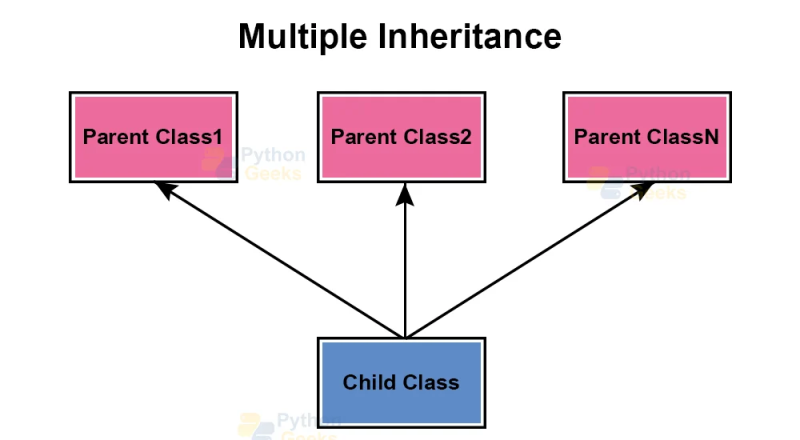``````class A:
def __init__(self, name):
self.name = name

def introduction(self):
print("我叫", self.name)

class B:
def __init__(self, age):
self.age = age

def introduction(self):
print("我今年 %d 岁了" % self.age)

class C(A, B):
pass

c = C(18)
c.introduction()
``````

``````我叫 18
``````

## 2.3 多级继承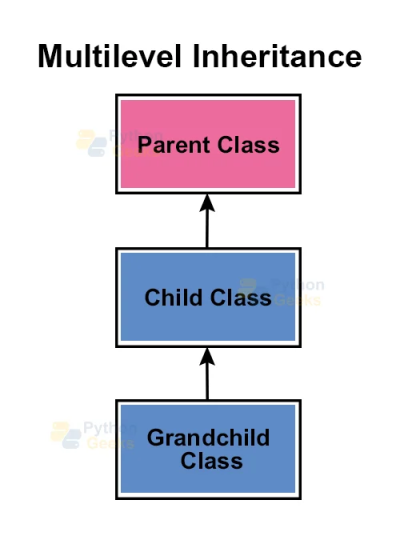``````class Parent:
str1 = "Python"

class Child(Parent):
str2 = " is the best programming?"

class GrandChild(Child):

def get_str(self):
print(self.str1 + self.str2)

person = GrandChild()
person.get_str()
``````

``````Python is the best programming?
``````

## 2.4 分层继承``````class SuperClass:
x = 2022

class SubClass1(SuperClass):
pass

class SubClass2(SuperClass):
pass

class SubClass3(SuperClass):
pass

a = SubClass1()
b = SubClass2()
c = SubClass3()
print(a.x, b.x, c.x)
``````

``````(2022, 2022, 2022)
``````

## 2.5 Python中的混合继承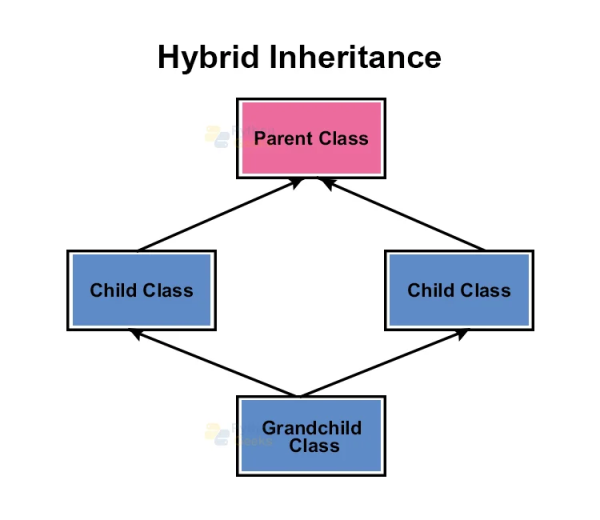``````class X:
num = 2023

class A(X):
pass

class B(A):
pass

class C(A):
pass

class D(B, C):
pass

ob = D()
print(D.num)
``````

``````2023
``````

# 3 总结

1帖子
视频
声望
粉丝
相关问题
社区精华内容